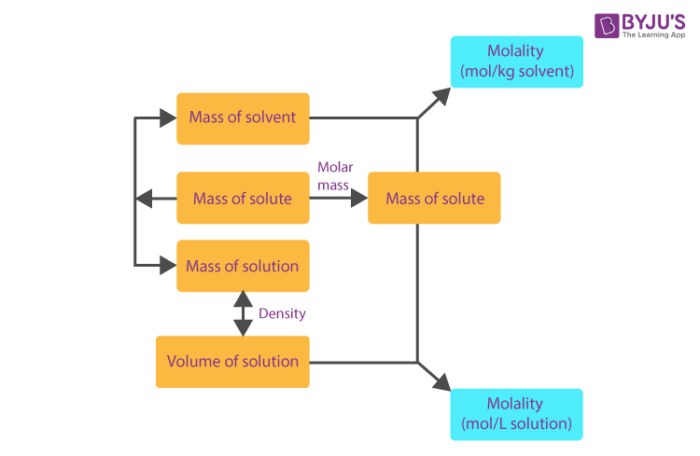Jet Set Go! All about Aeroplanes Jet Set Go! All about Aeroplanes

# Molarity And Mole Fraction

Majority of reactions happen in solutions and so it is important to understand how the amount of substance is expressed when it is present in the solution. There are many ways in which the amount of substances in solution is expressed:

## Molarity

It is one of the most widely used unit of concentration and is denoted by M. It is defined as no. of moles of solute present in 1 liter of solution. Thus,

$$\begin{array}{l}Molarity = \frac{No.~of~moles~of~solute}{Volume~of~solution(in~Litres)}\end{array}$$

## Mass Per Cent or weight percent (w/w %)

It is the ratio of the mass of solute to the mass of solution multiplied by 100 to calculate mass percent. It is also known as weight percent and is represented by (w/w %). You may have seen this symbol on the back of medicines and tablets. It is one of the most commonly used units of representing concentration.

Mathematically,

$$\begin{array}{l}Mass per cent =\frac{Mass~of~solute}{Mass~of~solution}~\times~100\end{array}$$

## Molality

It is defined as moles of solute present in 1-kilogram of solvent. It is denoted by m.

$$\begin{array}{l}Molality, m = \frac{No.~of~moles~of~solute}{Mass~ of ~solution(in~ kg)}\end{array}$$## Mole Fraction

The mole fraction or molar fraction (xi) is defined as the amount of a constituent (expressed in moles), ni, divided by the total amount of all constituents in a mixture (also expressed in moles), ntot:

$$\begin{array}{l}x_i=\frac{n_i}{n_{tot}}\end{array}$$

The sum of all the mole fractions is equal to 1:

$$\begin{array}{l}\sum_{i=1}^{N}n_i=n_{tot};\sum_{i=1}^{N}x_i=1\end{array}$$

#### An indicative example of Molarity

Question:– How much water should be added to 1 liter of 1 M KOH solution to make it 0.2 M KOH solution?

Solution:-

1M solution of KOH contains 1 mole of KOH in 1 liter of solution,

So, moles of KOH present in solution = 1 mole

Now we know,

$$\begin{array}{l}Molarity = \frac{No.~of~moles~of~solute}{Volume~of~solution(in~Litres)}\end{array}$$
$$\begin{array}{l}\Rightarrow~0.2= \frac{1}{x}\end{array}$$
$$\begin{array}{l}\Rightarrow~X = \frac{1}{0.2}\end{array}$$
$$\begin{array}{l}\Rightarrow~X = 5\end{array}$$

Hence, if 1 mole of a solution is present in 5 litres of solution, then the molarity of solution will be 0.2 M.

So, extra 4 litres of water should be added to the 1 liter of 1 M KOH solution to make it 0.2 M KOH solution.

## Frequently Asked Questions – FAQs

### What is molarity molality and mole fraction?

Molarity is the ratio of a solvent’s moles to a solution’s total litres. Both the solute and the solvent are part of the solution. Molality, on the other hand, is the ratio of the solute moles to the solvent kilogrammes. Mole fraction is the mole of a constituent divided by the total mole of all constituents in a mixture.

### How is mole fraction related to Molality?

The ratio of the number of moles of that component present in the solution to the total number of moles of all the solution components is known as the mole fraction of any component of a solution. Molality is known as the total solvent moles found in a solvent kilogramme.

### How do I calculate moles?

Use the molecular formula to find the molar mass; divide the mass of the compound by the molar mass of the compound, represented in grammes, to obtain the number of moles.

### How do you find molarity of HCl?

HCl moles are in a solution of 25.0 mL. Molarity is the ratio of solute moles and solution length. We can obtain the acid solution molarity by dividing the amount of moles of HCl by the volume (L) of the solution in which it was dissolved.

### What is molarity example?

You split the moles of solute by the litres of the solution to get the molarity. In a litre of solution, for example, a 0.25 mol/L NaOH solution contains 0.25 mol of sodium hydroxide. You need to know the number of solute moles and the total volume of the solution to determine the molarity of a solution.